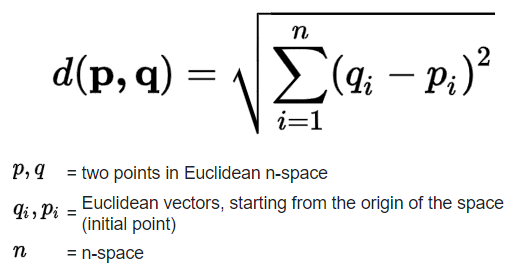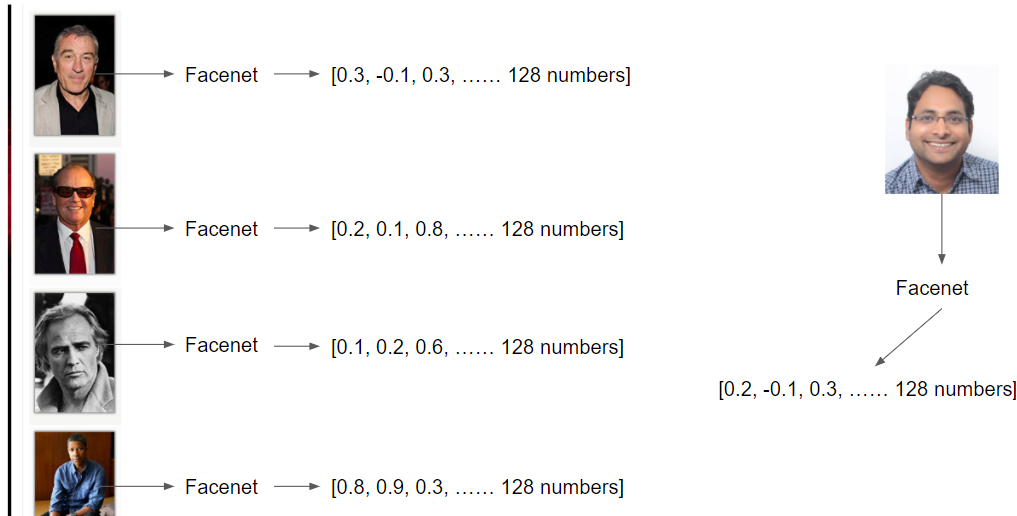# Celebrity Look-alike with Computer Vision - Calculate Euclidean Distance

Now, we would write a function that calculates the Euclidean distance between the face embeddings that we calculated in the previous steps. This is done to see how far apart the test image is from the known faces. It returns the distance that is minimum, and the path to that image.

What is Euclidean Distance?
The Euclidean distance (also known as the Pythagorean distance) between two points in Euclidean space is the length of a line segment between those two points. It can be calculated from the Cartesian coordinates of the points using the Pythagorean theorem. Given below is the formula to calculate the Euclidean distance between 2 points in n-dimensional space:Here, the `face_distance()` function compares a list of face encodings to a known face encoding and get a Euclidean distance for each comparison face. The distance tells you how similar the faces are.INSTRUCTIONS
1. Create a function named `calculate_face_distance()` as given below:

``````def calculate_face_distance(known_encodings, unknown_img_path, cutoff=0.5, num_results=4):
image_to_test_encoding = face_recognition.face_encodings(image_to_test)

face_distances = face_recognition.face_distance(known_encodings, image_to_test_encoding)
return (unknown_img_path, known_images[face_distances.argmin()])
``````

No hints are availble for this assesment

Answer is not availble for this assesment

Note - Having trouble with the assessment engine? Follow the steps listed here